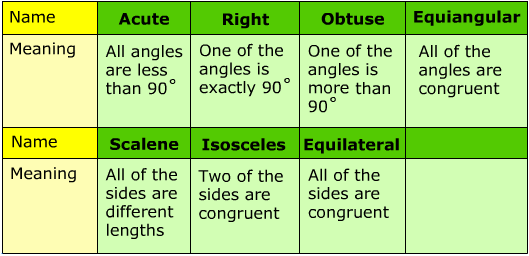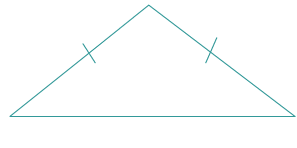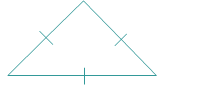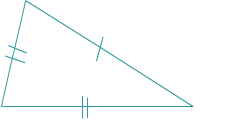# Naming Triangles

Triangles come in many shapes and sizes. When discussing the different types of triangles it is helpful to be able to name them.

There are two different types of names that can be given to a triangle.Use the chart below to help you as you investigate each triangle.Let's use the chart to work through some example.

1.) Name the triangle.Notice that there is a small box in the bottom corner. This means that the angle is 90°. Therefore, this is a right angle. It would appear that the sides are all different lengths. So we will also call this triangle scalene.

Therefore, this is a scalene right triangle.

2.) Name the triangle.Notice that this triangle has two slashes through the sides. This shows that these two sides are congruent. Two equal sides tells us that this triangle is isosceles. When two sides are congruent, there are also two angles congruent. The third angle on top is wider than 90°, otherwise known as obtuse.

Therefore, this is an obtuse isosceles triangle.

3.) Name the triangle.This triangle has three equal sides. We know this because of the dashed on the sides. When the sides are equal, the angles must be equal too. With 180 degrees in a triangle, each angle must be 60°. Because all of the angles measure less than 90°, we can also call this triangle acute.

Therefore, this triangle is an acute, equilateral and equiangular triangle. Most people just refer to this as an equilateral triangle.

4.)Name the triangle.The markings on this triangle show that the sides are not congruent. Therefore, this triangle is scalene. In addition, all three angles are less than 90°. That means that this triangle is also acute.

Therefore, we will call this an acute scalene triangle.

Let's Review

When naming a triangle, be sure to choose a name based on the side lengths and the angles. Look for markings that will give you information about whether the sides are congruent or the angles measure 90 degrees. Use this information to pick the best name possible.